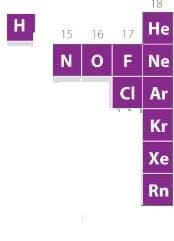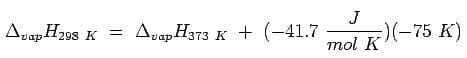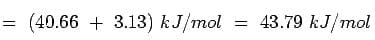JEE  >  Test: Effect of Heat on Matter

# Test: Effect of Heat on Matter

Test Description

## 20 Questions MCQ Test Chemistry Class 11 | Test: Effect of Heat on Matter

Test: Effect of Heat on Matter for JEE 2023 is part of Chemistry Class 11 preparation. The Test: Effect of Heat on Matter questions and answers have been prepared according to the JEE exam syllabus.The Test: Effect of Heat on Matter MCQs are made for JEE 2023 Exam. Find important definitions, questions, notes, meanings, examples, exercises, MCQs and online tests for Test: Effect of Heat on Matter below.
Solutions of Test: Effect of Heat on Matter questions in English are available as part of our Chemistry Class 11 for JEE & Test: Effect of Heat on Matter solutions in Hindi for Chemistry Class 11 course. Download more important topics, notes, lectures and mock test series for JEE Exam by signing up for free. Attempt Test: Effect of Heat on Matter | 20 questions in 20 minutes | Mock test for JEE preparation | Free important questions MCQ to study Chemistry Class 11 for JEE Exam | Download free PDF with solutions
 1 Crore+ students have signed up on EduRev. Have you?
Test: Effect of Heat on Matter - Question 1

### Among the following sentences the one that is false about gases is

Detailed Solution for Test: Effect of Heat on Matter - Question 1

Gasses are highly compressible because it is less dense than other states of matter. it's intermolecular force of attraction is less and it has more space between the molecules. Therefore, it is easy to compress gas.
While, solids are almost incompressible. This is because the intermolecular force of attraction in solids is greater compared to other states of matter. The moles in solids are tightly packed. Therefore, solids are almost incompressible.

Test: Effect of Heat on Matter - Question 2

### A cylinder of V litre capacity contains ammonia gas. This cylinder is inverted over another vessel of V litre capacity containing hydrogen chloride at the same temperature and pressure. The pressure in the cylinder after sometime will

Detailed Solution for Test: Effect of Heat on Matter - Question 2

As per Gay Lussac’s Law, when pressure and temperature are same V directly proportional to the moles. Let n moles of NH3 and n moles HCl after some time they react and form n moles NH4Cl and hence pressure drops.

Test: Effect of Heat on Matter - Question 3

### The number of elements that exists in gaseous state under normal atmospheric conditions is

Detailed Solution for Test: Effect of Heat on Matter - Question 3

A look at the periodic table shows us that there are 11 elements in the table that exist in the gaseous state at room temperature. These elements are Hydrogen, Helium, Nitrogen, Oxygen, Fluorine,  Chlorine, Neon, Argon, Krypton, Xenon, and Radon.Test: Effect of Heat on Matter - Question 4

The relation between Celsius scale and Fahrenheit scale of measuring temperature is

Detailed Solution for Test: Effect of Heat on Matter - Question 4

Invented in 1742 by the Swedish astronomer Anders Celsius, it is sometimes called the centigrade scale because of the 100-degree interval between the defined points. The following formula can be used to convert a temperature from its representation on the Fahrenheit ( F) scale to the Celsius (C) value: C = 5/9(F - 32).

Test: Effect of Heat on Matter - Question 5

Which one will have higher value of enthalpy of vaporization:

Detailed Solution for Test: Effect of Heat on Matter - Question 5

Higher value of enthalpy of vaporization means that we need to give high heat for the given liquid to vapour. So this is possible with only option b because with only option b, there is enough strong attractive force which we need to overcome by providing the most amount of heat in all the given cases.

Test: Effect of Heat on Matter - Question 6

The zero point temperature is:

Detailed Solution for Test: Effect of Heat on Matter - Question 6

It is obvious that a lower temperature is not feasible because there is no velocity smaller than zero and no energy content less than nothing. (As a side remark, energy in this instance means only the energy that can be taken away from the particles and does not include the rest mass or quantum mechanical zero-point energies for confined particles.) Absolute zero corresponds to –273 degrees Celsius and –460 degrees Fahrenheit.

Test: Effect of Heat on Matter - Question 7

Choose the characteristic property of gases from the following

Detailed Solution for Test: Effect of Heat on Matter - Question 7

Gases are not at all ridig as compared to solids and liquids  their intre particle arrangement are very far from each other. they do not have definet shape, size & volume.

Test: Effect of Heat on Matter - Question 8

Choose the one which is not a characteristic property of gases:

Detailed Solution for Test: Effect of Heat on Matter - Question 8

The Properties of Gases. Gases have three characteristic properties:
(1) they are easy to compress,
(2) they expand to fill their containers, and
(3) they occupy far more space than the liquids or solids from which they form.

Test: Effect of Heat on Matter - Question 9

Which of the following sublimes at ordinary temperature:

Detailed Solution for Test: Effect of Heat on Matter - Question 9

Camphor sublimes at ordinary temperature. It's a simple fact.

Test: Effect of Heat on Matter - Question 10

Thermal energy is responsible for:

Detailed Solution for Test: Effect of Heat on Matter - Question 10

Thermal energy refers to the energy contained within a system that is responsible for its temperature. Heat is the flow of thermal energy.Heat energy is the result of the movement of tiny particles called atoms,molecules or ions in solids, liquids and gases. Heat energy can be transferred from one object to another. The transfer or flow due to the difference in temperature between the two objects is called heat.

Test: Effect of Heat on Matter - Question 11

Thermal energy is directly proportional to:

Detailed Solution for Test: Effect of Heat on Matter - Question 11

Thermal energy is the sum of all the random kinetic energies of the molecules in a substance, that is, the energy in their motions. The higher the temperature, the greater the thermal energy. On the Kelvin temperature scale, thermal energy is directly proportional to temperature.

Test: Effect of Heat on Matter - Question 12

The conditions necessary for a gas to liquefy are

Detailed Solution for Test: Effect of Heat on Matter - Question 12

Liquiefing gas means changing of the substances in gaseous states are changed into liquid state.

To liquefy gas we must-

- Decrease temperature
- Increase pressure

By doing this the molecules of gas will come closer to each other and temperature is reduced so it will remove the enough energy to convert gas into liquid state. Thomas Andrews did work on liquefaction of gases like carbon dioxide.

Test: Effect of Heat on Matter - Question 13

The temperature in Celsius scale can be converted into Kelvin scale

Detailed Solution for Test: Effect of Heat on Matter - Question 13

To convert from Celsius to Kelvin you use the following formula:

Celsius temperature + 273.15 = Kelvin Temperature

For example:
26 °Celsius + 273.15 = 299.15 Kelvin

The Kelvin temperature scale was designed so that it starts at absolute zero. In Kelvin, absolute zero is equal to 0 degrees. In Celsius, absolute zero is equal to −273.15 degrees.

Therefore, you need to add 273.15 to the Celsius temperature to get to the Kelvin temperature.

Note: Kelvin does not use the degree symbol, °.

Test: Effect of Heat on Matter - Question 14

What happens to density of liquids on increasing temperatures:

Detailed Solution for Test: Effect of Heat on Matter - Question 14

Density increase as the temperature decreases. This is the reason why liquid water is more dense than solid water. The bonds in water break more slowly as temperature decreases and the structure tend to trap fewer extra water molecules. At low temperature, more of the water has the same lattice as ice.

Test: Effect of Heat on Matter - Question 15

What happens to boiling point of a liquid when pressure is increased:

Detailed Solution for Test: Effect of Heat on Matter - Question 15

The Boiling point of a liquid is the temperature at which the vapour pressure of the liquid equals the external pressure.

Also the vapour pressure of a liquid is a function of the temperature. As the temperature increases, the vapour pressure increases.

Test: Effect of Heat on Matter - Question 16

Sublimation involves the direct state change from:

Detailed Solution for Test: Effect of Heat on Matter - Question 16

Sublimation or volatilization is a change of state from solid to gas without passing through the liquid state. A typical example is dry ice, which can sublimate at r.t. and at atmospheric pressure.

Test: Effect of Heat on Matter - Question 17

Standard enthalpy of vaporization is taken at:

Detailed Solution for Test: Effect of Heat on Matter - Question 17

The standard enthalpy of vaporization of water at 298 K and 1 bar is thus:Test: Effect of Heat on Matter - Question 18

The standard boiling point of the liquid is

Detailed Solution for Test: Effect of Heat on Matter - Question 18

The standard boiling point is defined as the temperature at which boiling takes place under a pressure of 1 bar.

Whereas, the normal boiling point is defined as the temperature at which the vapour pressure of the liquid is equal to 1 atm at sea level.

Since 1 atm is equal to 1.01325 bar and hence is greater than 1 bar, the normal boiling point temperature is greater than the standard boiling point temperature.

Test: Effect of Heat on Matter - Question 19

Normal boiling point is

Detailed Solution for Test: Effect of Heat on Matter - Question 19

The boiling point of a liquid at 1 atm is also known as the normal boiling point. It has been found that Helium has the lowest normal boiling point (−268.9 °C) because it has very weak intermolecular attractions. Liquids that have high vapour pressures and low boiling points are known to be volatile, which means they evaporate quickly at room temperature. When the temperature increases, molecules start entering the gaseous state, consequently increasing the vapor pressure of the liquid until it comes into equilibrium with the atmospheric pressure.

Test: Effect of Heat on Matter - Question 20

In the process of conversion of a solid to liquid the average distance between the molecules is

Detailed Solution for Test: Effect of Heat on Matter - Question 20

The correct answer is option B
In ordered solids, the atomic spacing between two bonded atoms is generally around a few ångströms (Å), which is on the order of 10−10 meters. However, in very low density gasses (for example, in outer space) the average distance between atoms can be as large as a meter.

## Chemistry Class 11

204 videos|331 docs|229 tests
 Use Code STAYHOME200 and get INR 200 additional OFF Use Coupon Code
Information about Test: Effect of Heat on Matter Page
In this test you can find the Exam questions for Test: Effect of Heat on Matter solved & explained in the simplest way possible. Besides giving Questions and answers for Test: Effect of Heat on Matter, EduRev gives you an ample number of Online tests for practice

## Chemistry Class 11

204 videos|331 docs|229 tests